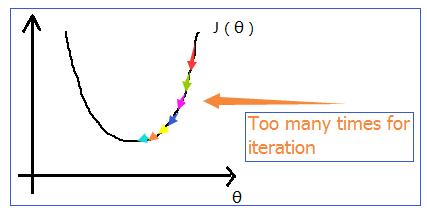# 机器学习笔记03：Normal equation与梯度下降的比较

《机器学习笔记02》中已经讲了多变量的梯度下降法，以及其他的一些小技巧和注意事项。下面来讲一种更加数学化的方法，我们称之为Normal equation，网上也没找到什么标准的翻译，就暂且称其为矩阵方程法吧。

# 一、简单回顾梯度下降# 二、Normal equation

1.当 θR $\theta\in R$ 时，误差函数为

J(θ)=aθ2+bθ+c

LetθJ(θ)=0

2.当 θRn+1 $\theta\in R^{n+1}$ 时，误差函数为
J(θ0,θ1,...,θn)=12mi=1m(hθ(x(i))y(i))2

LetθjJ(θ)=0(j=0,1,2,...,n)

Size( feet2 $feet^2$)Number of bedroomsNumber of floorsAge of house(years)Price( \$ $\$1000)
x0 $x_0$ x1 $x_1$ x2 $x_2$ x3 $x_3$ x4 $x_4$ y $y$
1 2104 5 1 45 460
1 1416 3 2 40 232
1 1534 3 2 30 315
1 852 2 1 36 178

X=11112104141615348525332122145403036;y=460232315178

x(i)1im=x(i)0x(i)1x(i)2...x(i)nR(n+1);X=(x(1))T(x(2))T(x(3))T...(x(m))T=x(1)0x(2)0x(3)0...x(m)0x(1)1x(2)1x(3)1...x(m)1x(1)2x(2)2x(3)2...x(m)2...............x(1)nx(2)nx(3)n...x(m)n;y=y(1)y(2)y(3)...y(m)

x(i)=[1x(i)1],X=1111x(1)1x(2)1...x(m)1;y=y(1)y(2)...y(m)

θ=θ0θ1θ2...θn

θ=(XTX)1XTy

1.单位矩阵 E $E$ 是一个对角线全为1，其他元素都为零的方阵 :
E=1000...00100...00010...00001...0..................0000...1

2.方阵 A $A$ 的逆矩阵记为 A1$A^{-1}$，同时其满足下面的特性（具体证明参考线性代数的相关书籍）:
A×A1=E
A1×A=E

y=Xθ

XTy=XTXθ

(XTX)1XTy=(XTX)1(XTX)θ

θ=(XTX)1XTy

# 三、梯度下降和Normal equation的比较

Needs to choose α $\alpha$ (需要选取合适的 α $\alpha$)No need to choose α $\alpha$ (不需要 α $\alpha$)
Needs many iterations (需要很多次的迭代计算)No need to iterate (不需要迭代计算)
Works well even when n is very large (在n很大时，能工作得很好)Needs to calculate (XTX)1 $(X^TX)^{-1}$ (需要计算 (XTX)1 $(X^TX)^{-1}$，其时间复杂度为 O(n3) $O(n^3)$)
Slow if n $n$ is very large (当n$n$非常大时，速度非常慢)

1.存在冗余特征。

x1 $x_1$ x2 $x_2$
meaningsize in feet2 $feet^2$size in m2 $m^2$

2.特征数量 n $n$ 过多，而训练样本数 m$m$ 过少。

——–转载请注明出处——–

11-27
09-1310-191538
03-2582
06-061万+
05-102万+
12-238505
07-13602
05-2041
03-204万+
11-255117